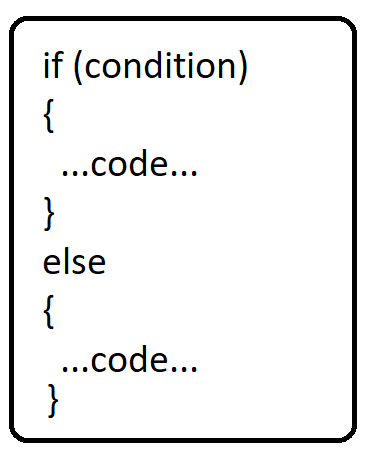# If – else in Java

### Introduction:

Let’s imagine you’re working on a project and that you have a requirement that some block of code has to be executed only when a condition is met, in this case, we will use if-else conditional statements

### Definition:

if-else statements can be defined as conditional statements because they are executed when a condition is met.

In this tutorial we will learn:

1. If statement
2. If-else
3. If – else if- else
4. Nested if-else### if statement

Executes code in the “if” block if the condition specified is true, otherwise, control of the program goes to the next line without executing code inside the “if” block.

Example :

Check whether a given number is strictly greater than 25

Code:

## Java Code

``````public class TUF {
public static void main(String[] args) {

int n = 50;

if (n > 25) {
System.out.println("Yes!");
}

}
}``````

Output: Yes!

Explanation: The condition in the “if” block is true ( 50 > 25), so the code in the “if” block is executed. If n is < 25, say 13 then code inside the “if” block will not be executed.

### if-else Statement

Execute code in the “if” block if the condition specified is true, otherwise execute the “else” part and then move on to the next lines.

Example:

Given the  age of a person check whether he/she is eligible for voting, print “Yes” if eligible else print “No”

Note: A person is eligible for voting if that person’s age is greater than 18

Code:

## Java Code

``````public class TUF {
public static void main(String[] args) {

int age = 34;

if (age > 18) {
System.out.println("Yes");
} else {
System.out.println("No");
}
}
}
``````

Output: Yes

### if – else if – else statement:

```1) if (condition)
2) {
3)       ….code…
4) }
5) else if (condition)
6) {
7)     ….code….
8) }
9) else
10){
11)   …code….
12) }

13) .......```

if” condition in line 1 is true ⇒ then code inside the “if” block will be executed and after execution, program control will go to line 13

if” condition in line 1 is false ⇒ then program control will go to the “else if”  part (line 5) and if the else if” part is true then it will execute, otherwise program control will go to the “else” part.

Example:

Code:

## Java Code

``````public class TUF {
public static void main(String[] args) {

if (10 > 20){  //false, so this will not execute
System.out.println("if statement executed");
}
else if ( 10 < 20 ) { //true, so this will execute
System.out.println("else if statement executed");
}
else {  // it will only execute when above statements are not executed
System.out.println("else statement executed ");
}
}
}
``````

Output:

else if statement executed

### Nested if-else

When we want to check for multiple conditions at a time and execute a specific code block, then we can use an if-else block inside an if block or nested if-else.

Example:

Problem statement: Given a number, we need to check if that number is divisible by 9. If it is divisible by 9, then we need to check if it’s even or odd and print “Divisible by 9 and even” or “Divisible by 9 and odd”. If it’s not divisible by 9, we print “Not divisible by 9”.

Here first we check if the number is divisible by 9. If it is divisible, then we check if it is even or odd.

Code:

## Java Code

``````public class TUF {
public static void main(String[] args) {

int n = 23;

if (n % 9 == 0) {
if (n % 2 == 0) {
System.out.println("Divisible by 9 and Even ");
} else {
System.out.println("Divisible by 9 and Odd ");
}
} else {
System.out.println("Not divisible by 9");
}
}
}
``````

Output:

Divisible by 9 and Even

Special thanks to Sai bargav Nellepalli for contributing to this article on takeUforward. If you also wish to share your knowledge with the takeUforward fam, please check out this article